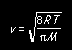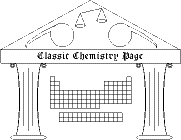## Bodenstein equilibrium

Max Bodenstein made detailed measurements of the thermodynamics and kinetics of the gas-phase decomposition of hydrogen iodide
2 HI < --- > H2 + I2 .
His work, published in 1899, was one of the first such investigations over an extended temperature range. He heated HI samples, waiting for the decomposition reaction to come to equilibrium at several temperatures. He analyzed the equilibrium mixutures and reported the fraction of HI that decomposed. His data are reproduced below.
 temp (°C) fraction decomposed, ξ 508 0.2408 487.2 0.2340 443 0.2198 427 0.2157 410 0.2100 393 0.2058 374 0.2010 356 0.1946 328 0.1885 302 0.1815 283 0.1787
1) The equilibrium constant is a function of the equilibrium pressures of the components,
K = pHpI/pHI2 .
Show that if the reaction mixture initially contained only HI at a pressure p, and if ξ denotes the fraction of HI that decomposed as the reaction proceded toward equilibrium, then the equilibrium constant can be expressed as
K = ξ2/[4(1 - ξ)2] .
2) Use the equation shown in exercise (1) to compute the equilibrium constant K at each temperature.

3) Make a van't Hoff plot (ln K vs. 1/T, where T is the absolute temperature) and report the best-fit straight line through the data. Use the plot to estimate the standard molar enthalpy and entropy changes (ΔH° and ΔS°) for the decomposition of HI.

### Reference

Max Bodenstein, "Gas reactions in chemical kinetics II. Effect of the temperature on the formation and decomposition of hydrogen iodide," Zeitschrift für physikalische Chemie 29, 295-314 (1899)
Copyright 2003 by Carmen Giunta. Permission is granted to reproduce for non-commercial educational purposes.Back to the Classic Calculations home pageBack to the top of the Classic Chemistry site